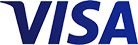Grid List
(Each)
(Each)
(Each)
(Each)
(Each)
(Each)
(Each)
(Each)
(Each)
(Each)
(Each)
(Each)